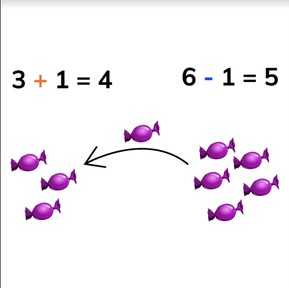Addition and Subtraction to 10 with 1 or 2

# Addition and Subtraction to 10 with 1 or 28,000 schools use Gynzy92,000 teachers use Gynzy1,600,000 students use Gynzy

## General

Students learn to add and subtract numbers to 10, in which they add or take away 1 or 2.

1.OA.D.6

## Relevance

It is important to be able to do this, to determine how many you have when you add to or take away 1 or 2.

## Introduction

Ask students to stand in a circle. Have students pass a ball around. The first student starts at 1, and the next counts one forward. Continue to 10 and then have students count backward back to 1.

## Development

Using the images on the interactive whiteboard, explain to students how to add using the flags. You count the total number of flags, drag one more to the row, and then count the total again. Explain to students that since you are adding one more, you count one more. Repeat this with demonstrating adding with the apples. Tell students that you do the same when adding two to a number. Then explain to students that you can also take 1 and 2 away. Show the fish in the pond. Count the fish, and then drag one fish into the bucket and then count the fish again. Repeat this explanation with the bottles. Tell students that you do the same when subtracting two from a number. To check that students are able to add and subtract one or two to a given number with visual support, ask them to solve the problems on the interactive whiteboard individually or in pairs. Finally show students that they can also solve these problems when just numbers are shown. Emphasize to students that you are adding by counting one or two forward, and subtracting by counting one or two backward.

To check that students understand how to add or subtract numbers to 10 with one or two, you can ask the following questions:
- What does + mean?
- What does - mean?
- What do you do if you want to know how much 5 + 1 is?
- What do you do if you want to know how much 8 - 2 is?

## Guided practice

Students are given subtraction problems with visual support that they can count. They are then given abstract problems with only numbers to solve. They are given addition and subtraction problems in which they add or subtract 1 or 2.

## Closing

Discuss the learning goal with the students and remind them that being able to add or subtract numbers to 10 by 1 or 2 is useful so you can figure out how many you have. The interactive whiteboard then shows a few slides with abstract math problems, with two kids who give answers. Ask students to determine which of the kids on the interactive whiteboard has the correct answer. Ask students to explain their strategy.

## Teaching tips

Students who have difficulty with this learning goal can be supported by the use of manipulatives, like blocks or tokens to use to physically add 1 or 2 or physically take 1 or 2 away.

## Instruction materials

Ball
Optional: blocks, tokens, or other manipulatives

### The online teaching platform for interactive whiteboards and displays in schools

• Save time building lessons

• Manage the classroom more efficiently

• Increase student engagement